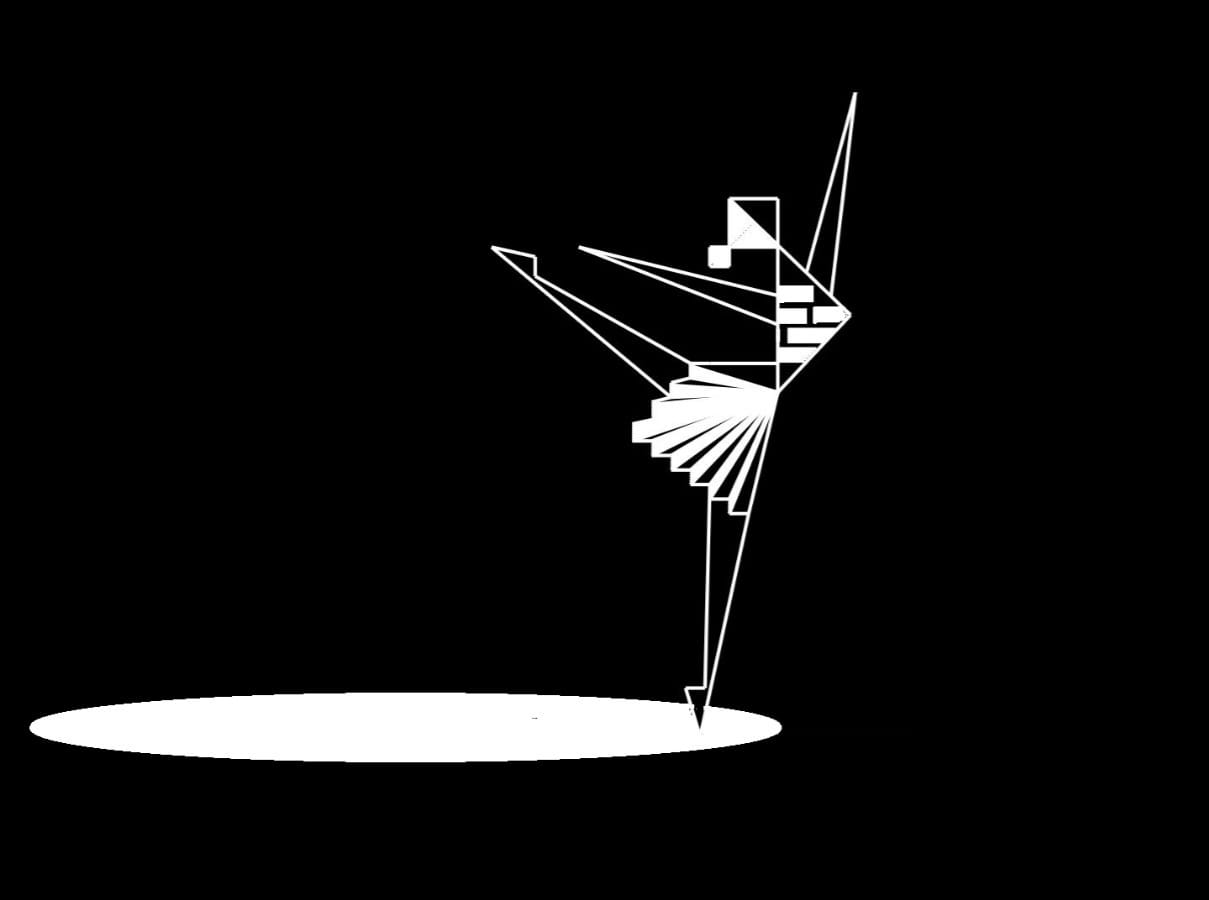## Mathematical and Algorithmic Art

This project explores the relationship between mathematics and art from different perspectives.

One aspect of this project involves the mathematization of shapes found in nature. Mathematical principles are used to understand and recreate the patterns and forms observed in nature as artistic representations. It also explores the evolution of shapes, investigating the transformative processes through which one shape can converge and transition into another.

Another aspect of the project is the generation of mathematical and algorithmic art. Mathematical formulae and notions such as lattices, fractals, and tessellations serve as the foundation for creating these artworks.

Presented here are selected artworks from this mathematical art project, made by Awansika Nimuthumana and Anuradha Mahasinghe.